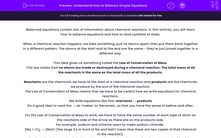# Understand How to Balance Simple Equations

In this worksheet, students will learn how to balance simple equations and how to use symbols of state.Key stage:  KS 4

Year:  GCSE

GCSE Boards:   AQA, AQA Trilogy, AQA Synergy, OCR 21st Century, OCR Gateway, Pearson Edexcel, Eduqas,

Difficulty level:#### Worksheet Overview

Balanced equations contain lots of information about chemical reactions. In this activity, you will learn how to balance equations and how to read symbols of state.

When a chemical reaction happens, we take something, pull its atoms apart, then put them back together in a different pattern. The atoms at the start and at the end are the same - they're just joined together in a different way.

This idea gives us something called the Law of Conservation of Mass.

This law states that no atoms are made or destroyed during a chemical reaction. The total mass of all the reactants is the same as the total mass of all the products.

Reactants are the chemicals we have at the start of a chemical reaction and products are the chemicals we produce by the end of the chemical reaction.

The Law of Conservation of Mass means that we have to be careful how we write equations for chemical reactions.

We write equations like this: reactants → products

It's a good idea to read the → as 'makes' or 'becomes', so that you have the sense of before and after.

For the Law of Conservation of Mass to work, we have to have the same number of each type of atom on the reactants side of the arrow as there are on the products side.

For example, sodium and chlorine react to make sodium chloride:

2Na + Cl2 → 2NaCl (the large 2's in front of Na and NaCl mean that there are two copies of that chemical in the reaction).

To check that the reaction is balanced, count the number of atoms of each type on each side of the reaction.

Na: before the reaction happens, there are 2Na, and after the reaction happens, there are 2Na.

Cl: before the reaction happens, there are 2Cl, and after the reaction happens, there are 2Cl.

No atoms have been made or destroyed, so the reaction is balanced.

If we need to balance an equation, we can't change the formulas of the chemicals we have, but we can change how many units of that chemical we use. So we were allowed to change NaCl to 2NaCl, but not to Na2Cl.

We can change the large numbers, but not the small ones.

Often, we add state symbols to each chemical, to tell us what the state the chemical is in. There are four symbols you need to know:

Symbol Meaning
(s) Solid
(l) Liquid
(g) Gas
(aq) Aqueous (dissolved in water)

Let's put all that together in an example.

When we burn methane gas, we react it with oxygen to make carbon dioxide and water. In symbols, the first guess at the equation is:

CH4 + O2 →CO2 + H2O but that equation isn't balanced. The carbons are balanced (one in CH4 on the reactants side, one in CO2 on the products side)

Look at the hydrogen atoms, though. There are four on the reactants side, in CH4. On the products side, there are only two, in H2O. We can't change H2O to H4O, but we can have two water molecules in the reaction. Now it looks like this:

CH4 + O2 →CO2 + 2H2O but that equation still isn't balanced.

Look at the oxygens. There are two on the reactants side in O2, and four on the products side (two in CO2 and two in the water molecules - one in each H2O). We can't change O2 to O4, but we can have two molecules of O2. So the balanced equation is:

CH4 + 2O2 →CO2 + 2H2O.

Methane, oxygen and carbon dioxide are all gases. Water is a liquid. So when we add the state symbols, we end up with:

CH4 (g) + 2O2 (g) →CO2 (g) + 2H2O (l).

If you're checking whether or not a reaction is balanced, count the atoms before and after for each element in turn. The Law of Conservation of Mass says that the numbers for each element have to be the same.

Remember that you can change the large numbers in front of the formulas, but not the small numbers in the formulas.

Now let's have a go at some questions.

### What is EdPlace?

We're your National Curriculum aligned online education content provider helping each child succeed in English, maths and science from year 1 to GCSE. With an EdPlace account you’ll be able to track and measure progress, helping each child achieve their best. We build confidence and attainment by personalising each child’s learning at a level that suits them.

Get started••••